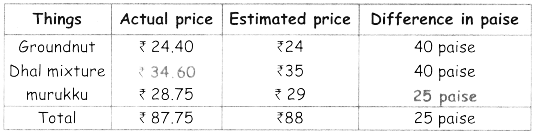Tamilnadu State Board New Syllabus Samacheer Kalvi 4th Maths Guide Pdf Term 3 Chapter 5 Money InText Questions Textbook Questions and Answers, Notes.

## Tamilnadu Samacheer Kalvi 4th Maths Solutions Term 3 Chapter 5 InText Questions

Denomination (Text Book Page No. 35)

Convert rupees to paise:
₹ 565 = _______ _______ _______ _______ _______ _______
₹ 200 × _______ =
₹ 100 × _______ =
₹ 50 × _______ =
₹ 10 × _______ =
₹ 5 × _______ =
Total = _______
₹ 565 = ₹ 200 ₹ 200 ₹ 100 ₹ 50 ₹ 10 ₹ 5
₹ 200 × 2= ₹ 400
₹ 100 × 1 = ₹ 100
₹ 50 × 1 = ₹ 50
₹ 10 × 1 = ₹ 10
₹ 5 × 1 = ₹ 5
Total = ₹ 265Try This (Text Book Page No. 36)Activity (Text Book Page No. 36)

Fill in the box with model notes.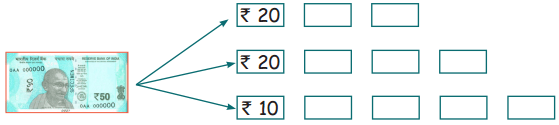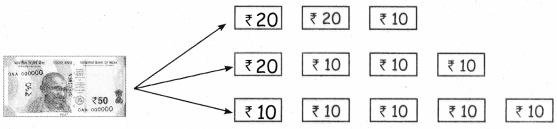Activity (Text Book Page No. 36)

Take a sheet of paper and cut out the model notes (₹ 10, ₹ 20, and ₹ 100). Affix it below the boxes provided to get the total of ₹ 200. One is done for you.Let us try (Text Book Page No. 41)

(i)(ii)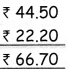(iii)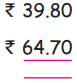(iv)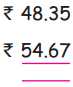Let us try (Add Book Page No. 41)

Subtract the following

(i)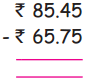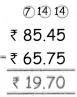(ii)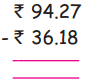(iii)(iv)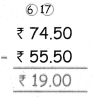Let us try (Add Book Page No. 44)

Find the cost per item, if the total cost is given. One is done for you.Fill in the following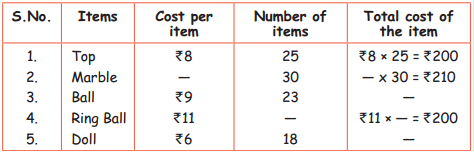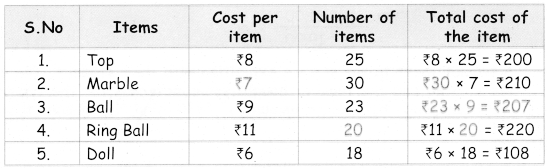Let us try (Add Book Page No. 47)

Find the estimated of the total cost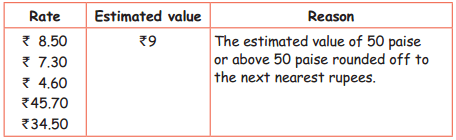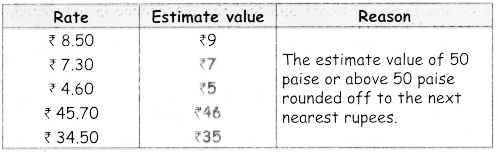Estimate the following rupees (before or after) using the multiples of ₹ 10.

 Actual price Estimated price ₹ 53 ₹ 50 ₹ 67 ₹ 48 ₹ 24 ₹ 97